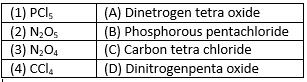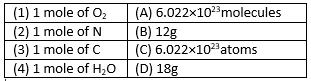Courses

# Test: Atoms And Molecules - 5

## 15 Questions MCQ Test General Science for UPSC (Civil Services) Prelims | Test: Atoms And Molecules - 5

Description
This mock test of Test: Atoms And Molecules - 5 for UPSC helps you for every UPSC entrance exam. This contains 15 Multiple Choice Questions for UPSC Test: Atoms And Molecules - 5 (mcq) to study with solutions a complete question bank. The solved questions answers in this Test: Atoms And Molecules - 5 quiz give you a good mix of easy questions and tough questions. UPSC students definitely take this Test: Atoms And Molecules - 5 exercise for a better result in the exam. You can find other Test: Atoms And Molecules - 5 extra questions, long questions & short questions for UPSC on EduRev as well by searching above.
QUESTION: 1

### Argentum is the Latin name of

Solution:

The Latin name of Silver is Argentum. The symbol of Silver is Ag.

QUESTION: 2

Solution:
QUESTION: 3

### What is the name given to 6.022 × 1023?

Solution:

Fixed number of atoms or molecules present in gram atomic mass of an atom or molecules is 6.022 × 1023.Thisnumber is known as Avogadro constant.

QUESTION: 4

Match the following with correct response.Solution:
QUESTION: 5

The number of moles for 52g of He is:
(Atomic Mass of He: 4u)

Solution:
QUESTION: 6

Which of the following is anion?
A. Magnesium
B. Sulphate
C. Oxalate
D. Ammonium

Solution:
QUESTION: 7

Calculate the number of particles in 0.01 mole of carbon atoms

Solution:

One mole of carbon contains6.022 × 1023atoms of carbon. The number of particles present in 0.01 mole of carbon = 6.022 × 1023×0.01=6.022 × 1021atoms.

QUESTION: 8

Match the following with correct response.Solution:
QUESTION: 9

Phosphorus is a

Solution:

Phosphorus is tetra-atomic. Each molecules of phosphorus contain 4 atoms.

QUESTION: 10

What is the ratio of magnesium and Sulphur by mass in Magnesium sulphide?

Solution:

Atomic mass of Magnesium is 12 and Sulphur is 16. Therefore, the ratio of Magnesium ans Sulphur by mass is 12:16 = 12/16 = 3:4.

QUESTION: 11

A sample of CaCO3 conatins 3.01×1023 ions of Ca2+ and (CO3)2-.The mass of the sample is

Solution:

The mass of one mole of CaCO3 is equal to 100 g. one mole of is equal to 6.022 × 1023ions. Therefore, mass of 3.01×1023 ions = 50 g

QUESTION: 12

Which is not true about H2SO4?
A. It is composed of 2 Hydrogen, 1 Sulpher and 4 Oxygen atoms
B. It relative molecular mass is 98
C. It is composed of one molecule of H2 One atom S and two molecules O2
D. Its relative molecular mass is 108

Solution:
QUESTION: 13

How many times an atom Sulphur is heavier than an atom of carbon?

Solution:

Atomic mass of Sulphur is 32 u and atomic mass of Carbon is 12 u. So, mass of Sulphur is 32/12 times heavier than Oxygen. = 8/3 times.

QUESTION: 14

“Gram atomic mass of an element and the gram molecular mass of a compound contains the same number of molecules” is a

Solution:

Gram atomic mass of an element and the gram molecular mass of a compound contain the same number of molecules, which is equal to6.022×1023molecules.

QUESTION: 15

Which of the following is correct definition of a mole?
A. It is equal to the gram atomic mass of the substance
B. It contain 6.022×1020 atoms of a given substance
C. It is equal to gram molecular mass of the substance
D. It contain 6.022×1023 molecules of a given substance

Solution: